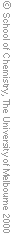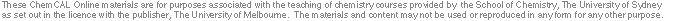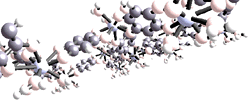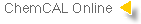1. General Chemistry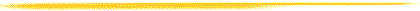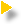Stoichiometry      The mole; balancing equations; stoichiometric calculations; molarity.Behaviour of Gases      The gas laws; ideal gas equation; real gases.States of Matter      Vapour pressure of liquids, phase diagrams and their interpretation.Electrochemistry      Oxidation numbers; redox equations; galvanic cells; electrode potentials.Chemical Energy and Calorimetry      Chemical energy; calorimetry; enthalpy; Hess's law; bond enthalpies.